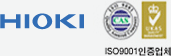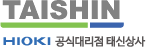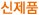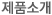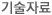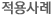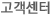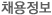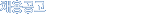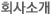검색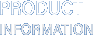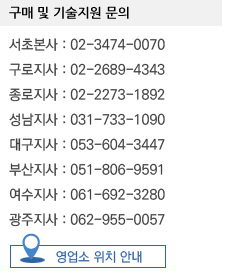47년 히오키 한국유통 태신상사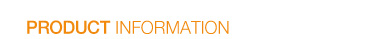HOME > 제품소개 > 제품정보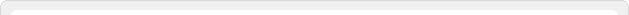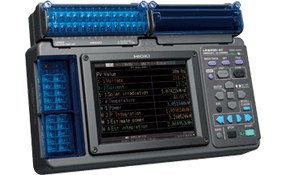LR8400-92, LR8400-93 추정 전력량을 계산하고 전력손실을 해결제품번호 : LR8400-92, LR8400-93제 품 명  : POWER VERIFIER제품분류 : 레코더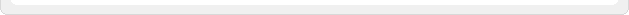* 본제품은 태신상사 기술영업사원과 상담이 필요합니다.
제품데모도 가능하오니 문의바랍니다.(문의처는 홈페이지 하단참조)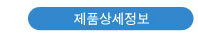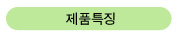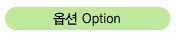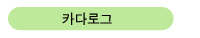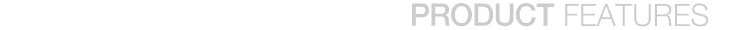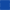적정한 발전량을 추정(추정 전력량) - 기온 및 일사량이 시시각각 변화하는 상황에서 지금 현재의 적정한 발전량을 추정 - 실제 발전량과의 추정치를 비교회로를 차단 시키지 않고 측정 가능시간경과에 따른 값의 변동 확인 가능차폐판(masking shield)과 함께 사용하면 쉽게 고장난 스트링과 모듈 확인가능PV 모드에서도 클램프 및 온도 등 최대 7ch의 추가 입력 가능(추가 클램프 필요)PV 모드 off시 30ch의 데이터 로거로 사용 가능PV 모드 off시 옵션 입력 장치를 추가하여 최대 60ch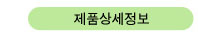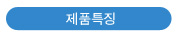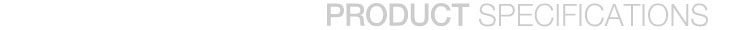PV mode Basic specifications
 Input (Fixed channels) (Automatic setting of conversion ratio) [unit1-ch1] Voltage measurement mode, 1 V f.s., 1,000 V conversion ratio [unit1-ch2] Current measurement mode, 1 V f.s., 1,000 A conversion ratio [unit1-ch3] Insolation measurement mode, 20 mV f.s., 140 kW/m2 conversion ratio [unit1-ch4] Panel temperature measurement mode, K thermocouple 100°C f.s., 1°C conversion ratio Expansion channel settings [unit1-ch5] to [unit1-ch11] Select and add as voltage, thermocouple, or clamp (2,000 A, 200 A, 100 A, 20 A, 10 A). *[unit1-ch12] to [unit1-ch15] and [unit2-ch1] to [unit2-ch15] cannot be used in PV mode. Equations (Fixed channels) (Automatic setting of equation) [W1] Power (kW) = Voltage (Ch. 1-1) × current (Ch. 1-2) [W2] Power integration (kWh) = Voltage (Ch. 1-1) × current (Ch. 1-2) × measurement time (h) [W3] Estimated power (kW) = Insolation strength (Ch. 1-3) / Gs (standard test condition of 1 kW/m2) × (1 + temperature loss*1) × (1 - coefficient for other losses) × photovoltaic cell rated output (kW) [W4] Estimated integration (kWh) = Insolation strength (Ch. 1-3) / Gs (standard test condition of 1 kW/m2) × (1 + temperature loss*1) × (1 - coefficient for other losses) × photovoltaic cell rated output (kW) × measurement time (h) *1 Temperature loss = Photovoltaic cell maximum output temperature coefficient × (panel temperature (Ch. 1-4) - 25) / 100 [W5] AC estimated power (kW) = Estimated power (w3) × power conditioner conversion efficiency (reference value) [W6] AC estimated integration (kWh) = Estimated energy (w4) × power conditioner conversion efficiency (reference value) [W7] Estimated power approximation rate (%) = Energy (w2) / estimated energy (w4) Display PV Wave + Value, PV Wave + Crsr, Gauge + PV Wave, PV Value, Estimate Power, AC Estimate (reference values) Other specifications Functionality is identical to that of the LR8400-20 when PV mode is OFF.

Applications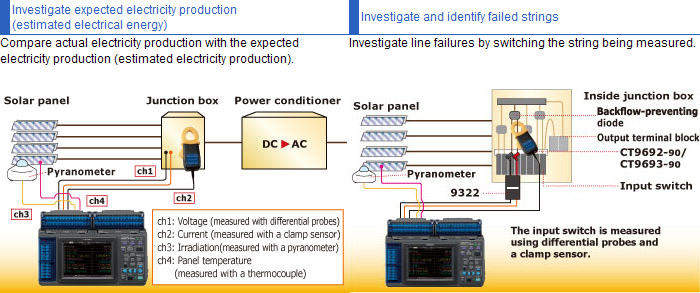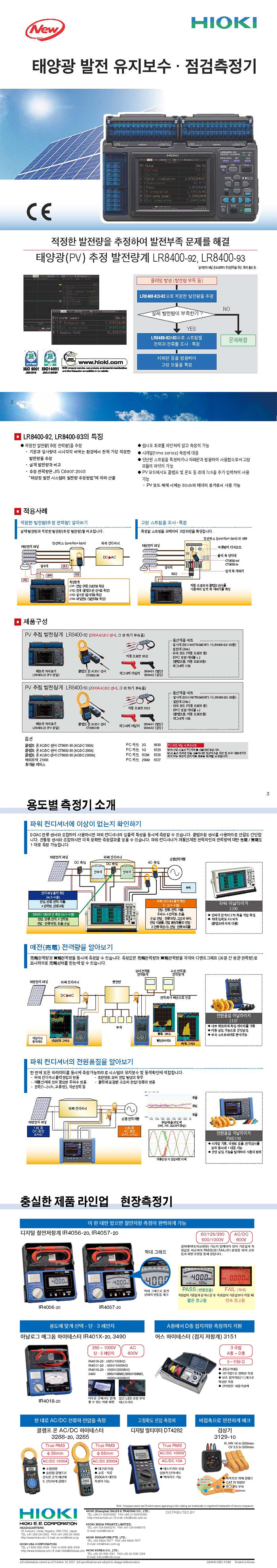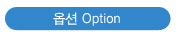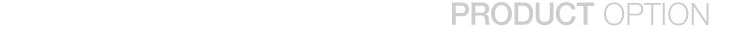Power supplies The AC adapter is standard equipment on the LR8400-92 and LR8400-93.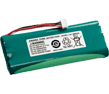BATTERY PACK
Z1000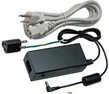9418-15

 PC communication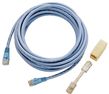LAN CABLE
9642

 Removable storage (CF card)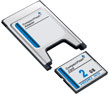PC CARD 2G
9830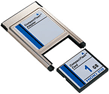PC CARD 1G
9729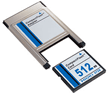PC CARD 512M
9728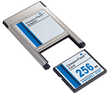PC CARD 256M
9727

 Current measurement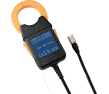CLAMP ON AC/DC SENSOR
CT9691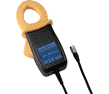CLAMP ON AC/DC SENSOR
CT9692CLAMP ON AC/DC SENSOR
CT9692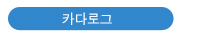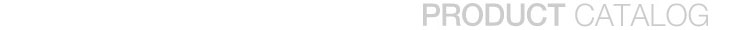서울특별시 서초구 서초동 1338-21 코리아비즈니스센터 1703호  T. 02-3474-0070  F. 02-3474-0090